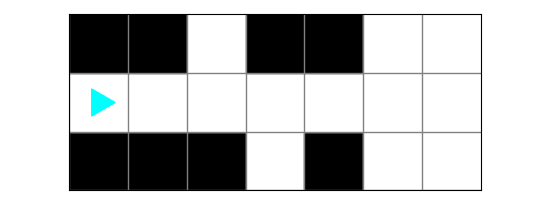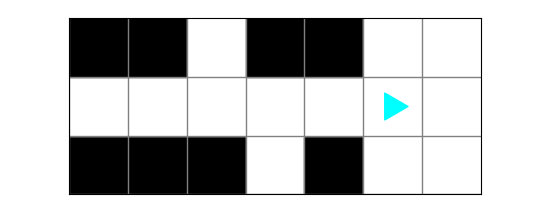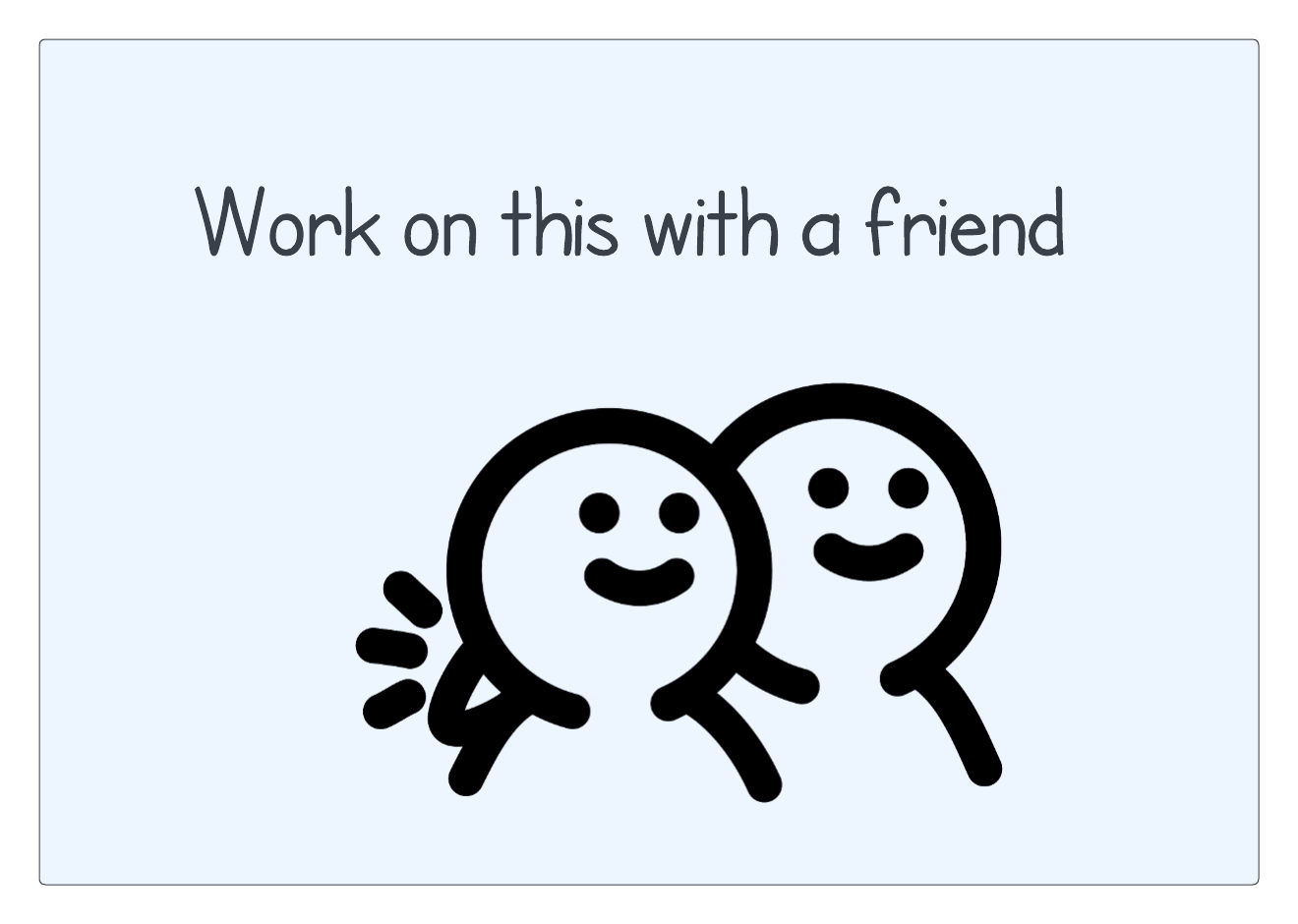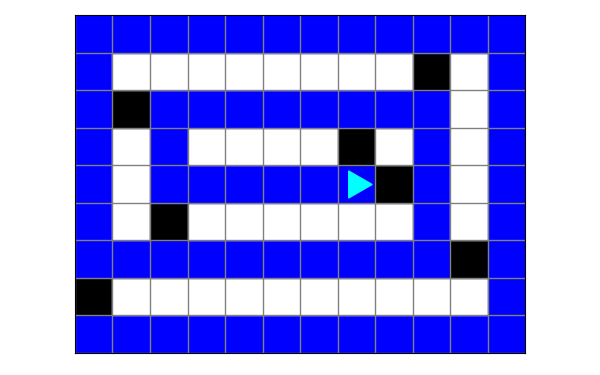Computer Science

# Practice with conditions

These problems will give you a chance to practice using `if` statements with conditions that include `and`, `or`, and `not`. Use the `event stream` pattern, and think carefully about what conditions you need.

Download the zip file above and put it in your `bit` folder.

## Freedom

For this problem, Bit starts in this world:Can you move Bit to the first space open on both sides? This is how the world should look when it is done:You can find some starter code in `freedom.py`:

``````from byubit import Bit

@Bit.worlds('freedom')
def go(bit):
# Write code here
pass

if __name__ == '__main__':
go(Bit.new_bit)``````

### Planning

When should Bit stop? What is the condition you would use? Express this in words, not Python code.There are two ways to think of this:

• Bit should move until the left is clear and the right is clear.
• Bit should move if the left is not clear or the right is not clear.

### Solution #1

Let’s start by looking at how the first solution would work — Bit should move until the left is clear and the right is clear.

You can write this with the following functions:

``````def found_freedom(bit):
return bit.left_clear() and bit.right_clear()

@Bit.worlds('freedom')
def go(bit):
bit.move()``````

We have put the stopping condition the left is clear and the right is clear into a function called `found_freedom()` that returns `True` if those conditions are met.

Then in `go()` Bit keeps moving `while not found_fredom(bit)`. In other words, Bit has to keep moving while the condition is not met.

Add this to your code and run it to see it working!

### Solution #2

Let’s now write code so that we follow the second suggestion, Bit should move if the left is not clear or the right is not clear. You can write this with the following functions:

``````def keep_moving(bit):
return not bit.left_clear() or not bit.right_clear()

@Bit.worlds('freedom')
def go(bit):
while keep_moving(bit):
bit.move()``````

Here, instead of a stopping condition we have keep moving condition. We write this in the function `keep_moving()` that returns `True` if either the left is not clear or the right is not clear.

Then in `go()` Bit keeps moving `while keep_moving(bit)`. In other words, Bit keeps moving while the condition is met.

Add this to your code and run it to see it working!

## Spiral

For this problem, Bit starts in this world:Can you move Bit in the shape of a spiral? This is how the world should look when it is done:You can find some starter code in `spiral.py`:

``````from byubit import Bit

@Bit.worlds('spiral')
def run(bit):
pass

if __name__ == '__main__':
run(Bit.new_bit)``````

### Planning

This problem looks more complex, but it can be simple if you focus on conditions. When should Bit stop? When should it turn left?An important thing to notice is that the spiral stops when Bit reaches a square where the front and the left are not clear.

1. The stopping condition for Bit is if the front is not clear and the left is not clear.

2. Bit should keep moving forward when it can, but turn left if the front is blocked.

### Solution

Here is one way to write these conditions:

``````def end_of_spiral(bit):
return not bit.front_clear() and not bit.left_clear()

@Bit.worlds('spiral')
def run(bit):
bit.paint('blue')
while not end_of_spiral(bit):
if not bit.front_clear():
bit.left()
bit.move()
bit.paint('blue')``````

We use the `end_of_spiral()` function to have the stopping condition. It stops if the front is not clear or the left is not clear.

We use the `run()` function to keep Bit moving and painting blue. We use `while not end_of_spiral(bit)` to keep Bit moving unless the stopping condition is met. Along the way, if the front is ever not clear, Bit turns left.

Notice that we also need an extra `bit.paint('blue')` to get the first square.

### An alternate solution

It’s important to recognize that there are multiple solutions to many problems! For example, you could instead write conditions like this:

1. Bit moves as long as the front is clear

2. If the front is ever not clear, Bit turns left.

Notice that if both the front and left are not clear, Bit will turn left, and then the front will be blocked. We need to be sure Bit doesn’t turn left again! That makes this solution a little more tricky to write.

Here is one way to do it:

``````@Bit.worlds('spiral')
def run(bit):
while bit.front_clear():
bit.paint('blue')
bit.move()
if not bit.front_clear():
bit.left()

bit.paint('blue')``````

Notice a few things that are different from the first solution. Bit needs to paint first, then move. At this point, if the front is not clear, Bit turns left. This way Bit can keep moving forward as long as the front is clear. But if it turns left and the front is blocked, it will stop and not turn again. Finally, we have to be sure the last square is painted blue, since Bit paints before it moves.